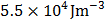# The locus of a point which moves such that the sum of the squares of its distance from three vertices of a triangle is constant is a/an a) Circle b) Straight line c) Ellipse d) None of these

## Question ID - 56055 :- The locus of a point which moves such that the sum of the squares of its distance from three vertices of a triangle is constant is a/an a) Circle b) Straight line c) Ellipse d) None of these

3537

(a)

Letandbe the vertices of the triangle, and letbe any point on the locus

Then,(constant)So, locus ofisWhereconstant

Next Question :
 The energy stored per unit volume in copper wire, which produces longitudinal strain of 0.1% isa)b)c)d)